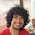### Break the sticks

Three question related to stick breaking. A stick is of length 1.

1) The stick drops and breaks at a random point distributed uniformly across the length. What is the expected length of the smaller part? (Source: DB interview)

2) In the above experiment, what is the expected length of the larger part? (Source: Made it up)

3) In the third experiment, the stick is dropped and breaks at two points. What is the probability that the three pieces could form a triangle? (Source: V.K.Bansal notes, MathOverflow.net)

Update (18/12/09): Solution: Partial solution by Aaditya Ramdas (CSE IITB 2005-2009) and Giridhar Addepalli (CSE, IITK alumnus and Yahoo! Sr. Software Engineer) and complete solution by me in comments.

1.ok, the first 2 parts are probably 0.25, and 0.75, and the third part seems to be some shady calculus thing (plot 3 inequalities n find the area of the region)...

2.u r right.. someone post the solution.. :)

3.Suppose stick is of length 1.
if all the 3 pieces are of length < 1/2 , subject to the constraint that sum of them is 1, then they form a triangle.

Two points are chosen uniformly,
Pr [ TF ] = Pr [TF | first point is in 1st half]
if a point belongs to [x , x+dx] , 0<=x<=1/2 , then the other point must belong to [1/2, 1/2+x] ( probability it belongs to this interval is x ).

[integral ( x dx ) 0 -> 1/2 ] / (1/2)
= 1/4.

4.@giridhar.. nice solution.. Thanx

5.1) The probability that the break point belongs to first half is 1/2. In this case, the expected length of the small part is L/4. Its L/4 in the other case too. Hence E = 1/2*L/4 + 1/2*L/4 = L/4 :) 0.25

2) Similarly, E = 3L/4. 0.75 .
This can also be seen by the fact that E1+E2=1. Hence, E2=0.75

3) Let the three parts be of the length x, y, 1-x-y
Feasible region: x,y>0 and x+y<1
Favourable region: x+y>1/2, x<1/2, y<1/2
So, the probability is 1/4 (One can explicitly see it if one draws the diagram) 0.25

6.Another Geometric solution ( i don't remember its source) :
Based on following FACT - The sum of the length of altitudes (to all 3 sides) from any point inside a equilateral triangle is equal to the Height of it.

In the question under consideration too, we had 3 lengths whose sum is 1.
So,let us consider the equilateral ABC triangle of height 1.

Now consider the triangle formed by joining the mid-points of the sides of ABC,call it DEF ;

Now observe that length of each altitude from any point inside DEF is less than 1/2. Hence satisfying the conditions forming a triangle.

If we consider altitudes from any point outside triangle DEF, one of them will have length > 1/2 .Hence we cannot have triangle with those lengths.

Area( DEF )/Area(ABC) = 1/4.

The above mentioned FACT is used in many other places, basically whenever we have 3 variables with constant sum.

I guess it can serve as a starting point for the "Empty Bucket" problem on this blog.

7.Nice.. very nice solution..
The proof of the FACT is trivial... did it in class VIII or IX.. :P

But the argument is strong.. thanx..

Although I am not able to see how this applies to empty bucket problem.. Help!!

8.join both ends of the stick to make a circle. Select 3 points on the circle (cutting points) . These 3 points would divide the stick in three parts. We are selecting 3 points at random. For that we first select 6 points (or 3 pairs of points with their antipodal points) and select a point from each pair. There are 2^3 ways to do this. Out of 8 only 2 will lead to the parts which can form triangle. If we choose 3 consecutive points out of 6 points we won't be able to make triangle.(and there are 6 ways to choose consecutive points). Hence the probability = 2/8 = 1/4. This argument can be used in many geometric probability puzzles like Probability of a random triangle on a circle (or in a disc) to lie in one half. Probability that center of a circle lies in a randomly chosen triangle on a circle. probability of a triangle on a circle being obtuse and many more.

For the Giridhar solution, there is a one to one correspondence between the lengths of the parts of stick and altitudes of the triangle. This must be true but it needs mathematical proof.